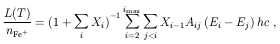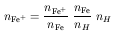4.5. Testcase #5

In this example we illustrate how PopRatio may be used to calculate the cooling rate due to collisional excitation of low-lying levels of a given atom or ion.

As an example we take the ion Fe+, because iron is an astrophysically abundant element. The ion Fe+ may be the dominating ionization stage in low ionization regions, and may be an important gas coolant .

The cooling rate is given by :(25)

Rewriting this in terms of the total Fe+ density nFe+ =i ni and the population ratios Xi = ni+1 / n1:(26)

with the energies Ei expressed in cm-1. The Fe+ density will depend on its fractional abundance and on the iron elemental abundance:(27)

Here we calculate the cooling function, defined as the right hand side of eq. (26).

Since Fe+ has a complicated electronic structure, several levels must be taken into account in the calculation. We employ a 16-level model ion, allowing us to calculate the cooling function for electronic densities as high as 104 cm-3.

To run this testcase the user does not need to modify function URAD, since no fluorescence transitions are loaded in.